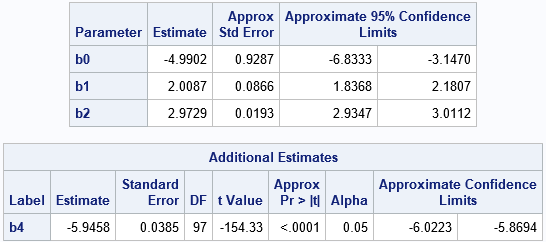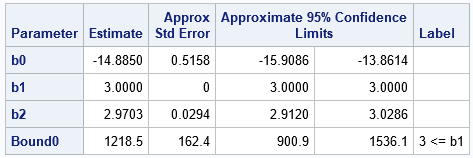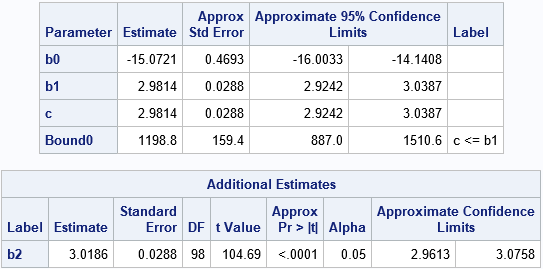A previous article discussed how to solve regression problems in which the parameters are constrained to be a specified constant (such as B1 = 1) or are restricted to obey a linear equation such as B4 = –2*B2. In SAS, you can use the RESTRICT statement in PROC REG to solve restricted least squares problems. However, if a constraint is an INEQUALITY instead of an EQUALITY, then (in general) a least squares method does not solve the problem. Therefore, you cannot use PROC REG to solve problems that have inequality constraints.

This article shows how to use PROC NLIN to solve linear regression problems whose regression coefficients are constrained by a linear inequality. Examples are B1 ≥ 3 or B1 + B2 ≥ 6.

### PROC NLIN and constrained regression problems

Before solving a problem that has inequality constraints, let's see how PROC NLIN solves a problem that has linear equality constraints. The following statements use the data and model from the previous article. The model is
Y = B0 + B1*X1 + B2*X2 + B3*X3 + B4*X4 + ε
where B3 = B1 and B4 = –2*B2. In PROC NLIN, you can replace the B3 and B4 parameters, thus leaving only three parameters in the model. If desired, you can use the ESTIMATE statement to recover the value of B4, as follows:

```/* You can solve the restricted problem by using PROC NLIN */ proc nlin data=RegSim noitprint; /* use NLINMEASURES to estimate MSE */ parameters b0=0 b1=3 b2=1; /* specify names of parameters and initial guess */ model Y = b0 + b1*x1 + b2*x2 + b1*x3 - 2*b2*x4; /* replace B3 = B1 and B4 = -2*B2 */ estimate 'b4' -2*b2; /* estimate B4 */ run;```The parameter estimates are the same as those produced by PROC REG in the previous article.

The syntax for PROC NLIN is natural and straightforward. The PARAMETERS statement specifies the names of the parameters in the problem; the other symbols (X1-X4, Y) refer to data set variables. You must specify an initial guess for each parameter. For nonlinear regressions, this can be a challenge, but there are some tricks you can use to choose a good initial guess. For LINEAR regressions, the initial guess shouldn't matter: you can use all zeros or all ones, if you want.

### Boundary constraints

Suppose that you want to force the regression coefficients to satisfy certain inequality constraints. You can use the BOUNDS statement in PROC NLIN to specify the constraints. The simplest type of constraint is to restrict a coefficient to a half-interval or interval. For example, the following call to PROC NLIN restricts B1 ≥ 3 and restricts B2 to the interval [0, 4].

```/* INEQUALITY constraints on the regression parameters */ proc nlin data=RegSim; parameters b0=0 b1=3 b2=1; /* initial guess */ bounds b1 >= 3, 0<= b2 <= 4; model Y = b0 + b1*x1 + b2*x2 + b1*x3 - 2*b2*x4; ods select ParameterEstimates; run;```The solution that minimizes the residual sum of squares (subject to the constraints) places the B1 parameter on the constraint B1=3. Therefore, a standard error is not available for that parameter estimate.

You can also use the BOUNDS statement to enforce simple relationships between parameters. For example, you can specify
bounds b1 >= b2;
to specify that the B1 coefficient must be greater than or equal to the B2 parameter.

### More general linear constraints

The BOUNDS statement is for simple relationships. For more complicated relationships, you might need to reparameterize the model. For example, the BOUNDS statement does not accept the following syntax:
bounds b1 >= 2*b2; /* INVALID SYNTAX! */
However, you can reparameterize the problem by introducing a new parameter C = 2*B2. You can then systematically substitute (C/2) everywhere that B2 appears. For example, the following statements show the constrained model in terms of the new parameter, C. You can use the ESTIMATE statement to find the estimates for the original parameter.

```/* let c = 2*b2 be the new parameter. Then substitute c/2 for b2 in the MODEL statement: */ proc nlin data=RegSim; parameters b0=0 b1=3 c=2; /* initial guess */ bounds b1 >= c; model Y = b0 + b1*x1 + (c/2)*x2 + b1*x3 - c*x4; estimate 'b2' c/2; /* estimate original parameter */ run;```

The output from this procedure is not shown.

You can use a similar trick to handle linear constraints such as B1 + B2 ≥ 6. Move the B2 term to the right side of the inequality and define C = 6 – B2. The constraint becomes B1 ≥ C and on the MODEL statement you can substitute (6–C) everywhere that B2 appears, as follows:

```/* Define c = 6 - b2 so that b2=6-c */ proc nlin data=RegSim; parameters b0=0 b1=5 c=5; bounds b1 >= c; model Y = b0 + b1*x1 + (6-c)*x2 + b1*x3 - 2*(6-c)*x4; estimate 'b2' 6-c; /* estimate original parameter */ ods select ParameterEstimates AdditionalEstimates; run;```### Summary

In summary, you can use the NLIN procedure to solve linear regression problems that have linear constraints among the coefficients. Each equality constraint enables you to eliminate one parameter in the MODEL statement. You can use the BOUNDS statement to specify simple inequality constraints. For more complicated constraints, you can reparametrize the model and again use the BOUNDS statement to specify the constraints. If desired, you can use the ESTIMATE statement to estimate the parameters in the original model.

Share